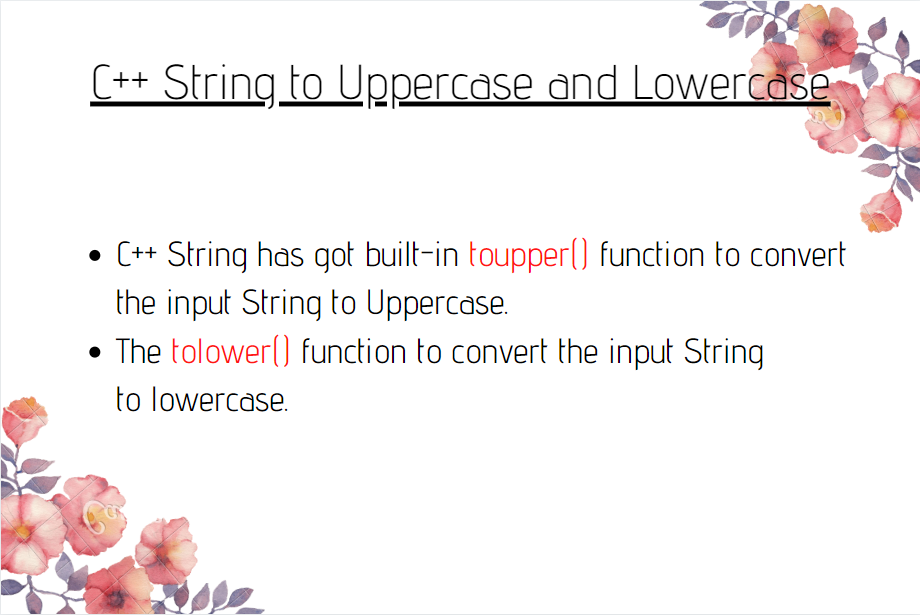# C++ String to Uppercase and Lowercase

Published on August 3, 2022By Safa Mulani
Developer and author at DigitalOcean.While we believe that this content benefits our community, we have not yet thoroughly reviewed it. If you have any suggestions for improvements, please let us know by clicking the “report an issue“ button at the bottom of the tutorial.

In this article, we will dive into the conversion of the input string to Lowercase and Uppercase in C++. C++ String class provides a huge number of built-in functions to perform operations over the input String.

## C++ String to Uppercase

C++ String has got built-in `toupper()` function to convert the input String to Uppercase.

Syntax:

``````toupper(input_string)
``````

Example:

``````#include <iostream>
#include <cstring>

using namespace std;

int main()
{
char arr[] = "Engineering Discipline.";

cout << "Original String:\n"<< arr<< endl;
cout<<"String in UPPERCASE:\n";
for (int x=0; x<strlen(arr); x++)
putchar(toupper(arr[x]));

return 0;
}
``````

In the above snippet of code, the cstring package contains the String related functions. Further, strlen() function is used to calculate the length of the input string.

The putchar() method is used to display the data on to the screen/console.

Output:

``````Original String:
Engineering Discipline.
String in UPPERCASE:
ENGINEERING DISCIPLINE.
``````

### Converting an input character to Uppercase

We can even convert the characters/string to Uppercase/Lowercase considering the ASCII values of the input characters.

ASCII values for lower case alphabets (a-z):97 - 122

ASCII values for upper case alphabets (A-Z):65 - 92

Example:

``````#include <iostream>
using namespace std;

int main()
{
char X;
cout<<"Enter a character:";
cin>>X;
X=X-32;
cout<<"Converted character to UPPERCASE:";
cout<<X;
return 0;
}
``````

As seen above, there happens to be a difference of 32 i.e. 97-65 between the range of ASCII values of lowercase and uppercase alphabets.

So in order to convert the input to uppercase, we need to subtract 32 from the ASCII value of the input character.

Output:

``````Enter a character:f
Converted character to UPPERCASE:F
``````

## C++ String to Lowercase

C++ String has got built-in `tolower()` function to convert the input string to lowercase.

Syntax:

``````tolower(input)
``````

Example:

``````#include <iostream>
#include <cstring>

using namespace std;

int main()
{
char arr[] = "Engineering Discipline.";

cout << "Original String:\n"<< arr<< endl;
cout<<"String in lowercase:\n";
for (int x=0; x<strlen(arr); x++)
putchar(tolower(arr[x]));

return 0;
}
``````

Output:

``````Original String:
Engineering Discipline.
String in lowercase:
engineering discipline.
``````

### Converting an input character to Lowercase

Example:

``````#include <iostream>
using namespace std;

int main()
{
char X;
cout<<"Enter a character:";
cin>>X;
X=X+32;
cout<<"Converted character to lowercase:";
cout<<X;
return 0;
}
``````

We need to add 32 to the ASCII value of the input character to convert it to lowercase.

Output:

``````Enter a character:R
Converted character to lowercase:r
``````

## Conclusion

In this article, we have understood the conversion of character and String input to Lowercase and Uppercase in C++. The important thing to note with the ASCII methods is the fact that they’re simply converting the entered characters to ASCII and then back. If someone enters a number instead of a character, you’ll get a random output.

So you can either handle the inputs and make sure that the entered values are actually characters, or simply use the toupper() and tolower() functions. We hope this tutorial has been useful to you. Comment below if you have any questions.